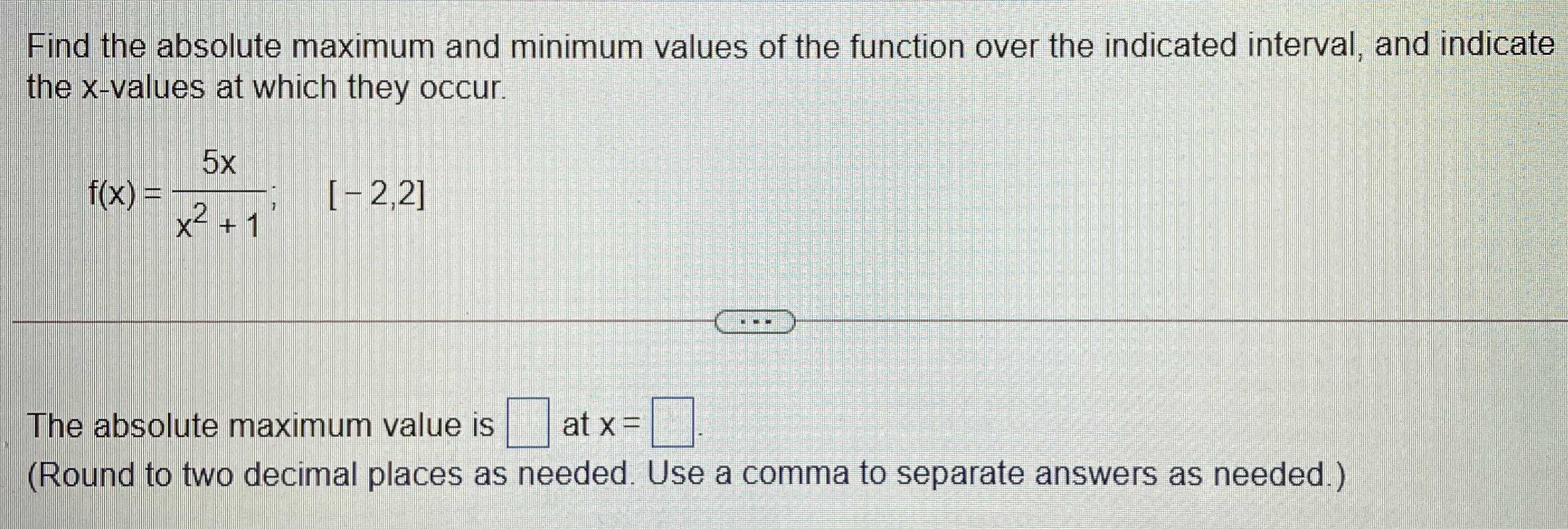### Still have math questions?

Algebra
QuestionFind the absolute maximum and minimum values of the function over the indicated interval, and indicate the $$x$$ -values at which they occur.

$$f ( x ) = \frac { 5 x } { x ^ { 2 } + 1 } ; [ - 2,2 ]$$

The absolute maximum value is $$\square$$ at $$x = \square$$ . (Round to two decimal places as needed. Use a comma to separate answers as needed.)

$$2.50,1.00$$